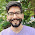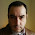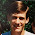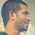VHDL coding tips and tricks: How to implement State machines in VHDL?

## Monday, April 26, 2010

### How to implement State machines in VHDL?

A finite state machine (FSM) or simply a state machine, is a model of behavior composed of a finite number of states, transitions between those states, and actions.It is like a "flow graph" where we can see how the logic runs when certain conditions are met.
In this aricle I have implemented a Mealy type state machine in VHDL.The state machine bubble diagram in the below figure shows the operation of a four-state machine that reacts to a single input "input" as well as previous-state conditions.
The code is given below:

library ieee;
use IEEE.std_logic_1164.all;

entity mealy is
port (clk : in std_logic;
reset : in std_logic;
input : in std_logic;
output : out std_logic
);
end mealy;

architecture behavioral of mealy is

type state_type is (s0,s1,s2,s3);  --type of state machine.
signal current_s,next_s: state_type;  --current and next state declaration.

begin

process (clk,reset)
begin
if (reset='1') then
current_s <= s0;  --default state on reset.
elsif (rising_edge(clk)) then
current_s <= next_s;   --state change.
end if;
end process;

--state machine process.
process (current_s,input)
begin
case current_s is
when s0 =>        --when current state is "s0"
if(input ='0') then
output <= '0';
next_s <= s1;
else
output <= '1';
next_s <= s2;
end if;

when s1 =>;        --when current state is "s1"
if(input ='0') then
output <= '0';
next_s <s3;
else
output <= '0';
next_s <= s1;
end if;

when s2 =>       --when current state is "s2"
if(input ='0') then
output <= '1';
next_s <= s2;
else
output <= '0';
next_s <= s3;
end if;

when s3 =>         --when current state is "s3"
if(input ='0') then
output <= '1';
next_s <= s3;
else
output <'1';
next_s <= s0;
end if;
end case;
end process;

end behavioral;

I think the code is self explanatory.Depending upon the input and current state the next state is changed.And at the rising edge of the clock, current state is made equal to next state.A "case" statement is used for jumping between states.
The code was synthesised using Xilinx XST and the results are shown below:

---------------------------------------------------------

States                      4
Transitions                 8
Inputs                      1
Outputs                     4
Clock                       clk (rising_edge)
Reset                       reset (positive)
Reset type                  asynchronous
Reset State                 s0
Power Up State              s0
Encoding                    Automatic
Implementation              LUT

---------------------------------------------------------

Optimizing FSM on signal with Automatic encoding.
-------------------
State | Encoding
-------------------
s0    | 00
s1    | 01
s2    | 11
s3    | 10
-------------------

Minimum period: 0.926ns (Maximum Frequency: 1080.030MHz)
Minimum input arrival time before clock: 1.337ns
Maximum output required time after clock: 3.305ns
Maximum combinational path delay: 3.716ns

The technology schematic is shown below:

As you can see from the schematic, XST has used two flipflops for implementing the state machine.The design can be implemented in hardware using many FSM encoding algorithms.The algorithm used here is "Auto" which selects the needed optimization algorithms during the synthesis process.Similarly there are other algorithms like one-hot,compact,gray,sequential,Johnson,speed1 etc.The required algorithm can be selected by going to Process -> Properties -> HDL options -> FSM encoding algorithm in the main menu.Now select the required one, from the drop down list.
More information about these options can be found here.

A very popular encoding method for FSM is One-Hot, where only one state variable bit is set, or "hot," for each state.The synthesis details for the above state machine implementation using One-hot method is given below:

Optimizing FSM on signal with one-hot encoding.
-------------------
State | Encoding
-------------------
s0    | 0001
s1    | 0010
s2    | 0100
s3    | 1000
-------------------

Minimum period: 1.035ns (Maximum Frequency: 966.464MHz)
Minimum input arrival time before clock: 1.407ns
Maximum output required time after clock: 3.418ns
Maximum combinational path delay: 3.786ns

The Technology schematic is shown below:
The main disadvantage of One-hot encoding method can be seen from the schematic.It uses 4 flip flops while, binary coding which is explained in the beginning of this article, uses only 2 flip flops.In general, for implementing a (2^n) state machine , binary method take n-flip flops while one hot method takes (2^n) flip flops.
But there are some advantages with one-hot method:
1)Because only two bits change per transition, power consumption is small.
2)They are easy to implement in schematics.

#### 23 comments:

1.Can you please clarify what does &lt and &gt stand for in code ?

1.It stands for less than and greater than

2.@melenith: sorry for the mistake.That was a problem with the syntax highlighter I have used.I have fixed it.Please check again.

Thanks for pointing out the mistake.

3.very nice blog! keep up the good work :)

4.When I write VHDL or Verilog I like to use automated tools. C-to-Verilog.com is a website which allows you to compiler your C code into hardware circuits. It has a great pipelining algorithm for superb performance.

5.Hi,

thanks for all the explanations, great work!

Do you know if it's possible to create some of the "Technology schematic" Graphs from the commandline? Something which could work out of a Makefile, perhaps...

6.Hi, guys!

I have to make a vhdl project to my engineering course...

Can anybody help me, with suggestions?

I even don't know what vhdl is exactly able to do...

Tks!

7.State machines are easier to design, maintain, etc. when kept in a single process, and are also less error-prone.

1.I'm coming quite late to the party here but how is it separating the sequential from the combinational logic more error prone. The split is intrinsic to a Moore machine, for example, why shouldn't this split be reflected in VHDL?

2.This comment has been removed by the author.

8.@foam : I do agree. See this post. I have did it with a single process.
http://vhdlguru.blogspot.com/search/label/state%20machine

9.can i get one hot code for FSM using 9 state flip flops in behavioral and structural

10.@raj : Please see the above example and code it yourself. I only do customized codes for a fee.

11.This comment has been removed by the author.

12.Can u plz post testbench code for this fsm.
Thanks

13.Why can't you just use one signal to remember the state instead of two? Like signal_state instead of current_s and next_s, and assign the next state according the current value of signal_state.

What is the difference? Would you care to explain?

Thank you very much...

14.Can you explain the state machine too.

15.You have an extra semicolon on this line

when s1 =>; --when current state is "s1"

16.sir how can we give delay in a program other than using wait statement

17.Is there a way to convert this to web and make it interactive using CSS?

Regards,
Creately

18.Can you do custom encoding in the actual VHDL code?

19.Just to improve a bit more on how the state machines gets translated into hardware , here a diagram :
https://ibb.co/nQmR8F

20.Your code does not simulate properly